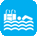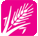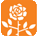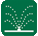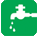HomeTechnical Information › Useful Conversions### Useful Conversionss » Conversion Calculators

Who needs to know anything about pumps? You do!

Mechanical pumps are the second most common machine in the world, after electric motors.

### Metric to U.S. System Conversions, Calculations, Equations and Formulas:

• Millimeters (mm) x 0.03937 = inches (")(in)
• Centimeters (cm) x 0.3937 = inches (")(in)
• Meters (m) x 39.37 = inches (")(in)
• Meters (m) x 3.281 = feet (')(ft)
• Meters (m) x 1.094 = yards (yds)
• Kilometers (km) x 0.62137 = miles (mi)
• Kilometers (km) x 3280.87 = feet (')(ft)
• Liters (l) x 0.2642 = gallons (U.S.)(gals)
• Liters (l) x 0.0353 = cubic feet
• Bars x 14.5038 = pounds per square inch (PSI)
• Kilograms (kg) x 2.205 = Pounds (P)
• Kilometers (km) x 1093.62 = yards (yds)
• Square centimeters x 0.155 = square inches
• Square meters x 10.76 = square feet
• Square kilometers x 0.386 = square miles
• Cubic centimeters x 0.06102 = cubic inches
• Cubic meters x 35.315 = cubic feet

### U.S. to Metric Conversions, Formulas, Calculations and Equations:

• Inches (")(in) x 25.4 = millimeters (mm)
• Inches (")(in) x 2.54 = centimeters (cm)
• Inches (")(in) x 0.0254 = meters (m)
• Feet (')(ft) x 0.3048 = meters (m)
• Yards (yds) x 0.9144 = meters (m)
• Miles (mi) x 1.6093 = kilometers (km)
• Feet (')(ft) x 0.0003048 = kilometers (km)
• Gallons (gals) x 3.78 = liters (l)
• Cubic feet x 28.316 = liters (l)
• Pounds (P) x 0.4536 = kilograms (kg)
• Square inches x 6.452 = square centimeters
• Square feet x 0.0929 = square meters
• Square miles x 2.59 = square kilometers
• Acres x 4046.85 = square meters
• Cubic inches x 16.39 = cubic centimeters
• Cubic feet x 0.0283 = cubic meters

### Area and Distance Conversions, Equations, Calculations and Formulas:

• Acre x 43560 = square feet
• Inches x 0.0833 = feet
• Feet x 12 = inches
• Square mile ("Sections") x 640 = Acres
• Miles x 5280 = feet
• Circumference of circle x 0.3183 = Diameter of the circle
• Diameter of circle x 3.14 = Circumference of circle
• Diameter squared x 0.7854 = Area of circle
• Radius squared x 3.14 = Area of circle
• Miles x 1760 = yards
• Square Miles x 259 = hectares
• Square kilometers x 100 = hectares
• Acres x 40.47 = areas
• Hectares x 100 = areas
• Areas x 100 = square meters
• Yards x 3 = feet
• Acres x 4840 = square yards

### Water Pressure Equations, Conversions, Formulas and Calculations:

• Feet head (of water) x .433 = Pounds per square inch (PSI)
• Feet head (of water) x 0.3049 = meters head (of water)
• Feet head (of water) x 0.0295 = atmospheres
• Feet head (of water) x 2.988 = kiloPascals
• Meters head (of water) x 3.28 = feet head (of water)
• atmosphere (Atm) x 1.013 = 1 bar (b)
• atmosphere x 14.696 = Pounds per square inch (PSI)
• bars x 1.033 = kilograms per square centimeter
• bars x 14.67 = Pounds per square inch (PSI)
• bars x 100 = kiloPascals
• Kilograms per square centimeter x 14.223 = pounds per square inch (PSI)
• Pounds per square inch (PSI) x 2.31 = feet head (ft. hd.)
• Pounds per square inch (PSI) x 0.0703 = kilograms per square centimeter
• Pounds per square inch (PSI) x 0.06895 = bars
• Pounds per square inch (PSI) x 0.06805 = atmospheres
• Pounds per square inch (PSI) x 2.31 = feet head (of water)
• Pounds per square inch (PSI) x 6.896 = kiloPascals
• kiloPascal x 0.145 = Pounds per square inch (PSI)
• kiloPascal x 0.01 = bars
• kiloPascal x 0.334 = feet head (of water)

### Flow and Water Volume Formulas, Conversions, Calculations and Equations:

• U.S. Gallons per minute (gpm) x .1337 = Cubic feet per minute
• Cubic feet per minute x 7.48 = U.S. gallons per minute
• Cubic feet per second x 448.8 = U.S. gallons per minute
• U.S. gallons per minute x 0.00223 = Cubic feet per second
• Acre inches per hour x 453 = U.S. gallons per minute
• British Imperial gallons x 1.201 = U.S. gallons
• U.S. gallons x 0.833 = British Imperial gallons
• Acre feet x 325,850 = U.S. gallons
• Acre inches x 27154 = U.S. gallons
• Velocity in feet per second = (0.408 x GPM) / Inside diameter of pipe in inches, squared
• Q=AV (quantity = area x velocity) ("the basic equation of water flow")
(example: quantity in cubic feet per second = square feet of area x feet per second velocity)
• One inch of water depth = 0.62 gallons per square foot of area
• GPM x 226.8 = liters per hour
• cubic feet per minute x 1699 = liters per hour
• acre inches per hour x 1712.3 = liters per minute
• acre feet x 1231.7 = cubic meters
• acre inch x 102.64 = cubic meters
• velocity (feet/second) x 0.3047 = velocity (meters/second)
• velocity (meters/second) x 3.281 = velocity (feet/second)

### Pump Calculations, Conversions, Equations and Formulas:

The following formulas assume 55% pump efficiency (the standard assumption).

Note: Horsepower is Brake horse power for an electric motor. Do not use for fuel-powered pump engines!

• GPM = (horsepower x 2178) / feet head
• Feet head = (2178 x horsepower) / GPM
• Efficiency of pump = (GPM x feet head) / (horsepower x 3960)
• Horse powers x 745.7 = watts (W)
• Water flow (in cubic meters per second) = 0.55 x pump power (watts) / pressure (pascals)
• Water flow (in liters per second) = 5.43 x pump power (kilowatts) / pressure (bars)

### Miscellaneous Irrigation Formulas, Conversions, Equations and Calculations:

Sprinkler Precipitation Rate:
Note: "head spacing" is the distance between sprinkler heads. L = length between heads in the row, W = width between rows. Therefore "head spacing " = L x W. Use the GPM value for a full circle sprinkler. So if your sprinklers are all half circles, you need to double the GPM value so it is equivalent to a full circle value.

1. Precipitation rate for square sprinkler spacing:
GPM of full circle sprinkler x 96.3) / head spacing in feet = precipitation rate in inches per hour
2. Precipitation rate for triangular sprinkler spacing:
(GPM of full circle sprinkler x 96.3) / (head spacing in feet x 0.866) = precipitation rate in inches per hour

Example: Rotors arranged in rows. The rows are 25 feet apart and the sprinklers are 30 feet apart in the row. The heads are arranged in a triangle pattern. The heads use 6 GPM.

(6 x 96.3) / [(25 x 30) x 0.866]

577.8 /  x 0.866

577.8 / 649.5

0.89 inches per hour

### Friction Loss Calculators:

• Friction loss calculations are for standard PVC pipe.
• Cl 160 PVC pipe is not commonly available in 1/2" size, therefore the calculator assumes that Cl 315 pipe will be substituted.
• Cl 200 PVC pipe is not commonly available in 1/2" size, therefore the calculator assumes that Cl 315 pipe will be substituted.
• Cl 160 PVC pipe is not commonly available in 3/4" size, therefore the calculator assumes that Cl 200 pipe will be substituted.
• This calculator uses a contracted form of the Hazen-Williams formula which is the industry standard for calculating friction loss.
• A conservative C coefficient of 140 is used for PVC pipe (many authorities use 150 which is risky as it leaves no margin for error).
• Velocities over 7 feet per second should not be allowed in PVC pipe.
• Velocities over 5 feet per second should be avoided, especially with pressures higher than 80 PSI, or in pipe larger than 2".
• See Live Calculation